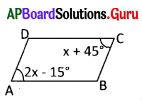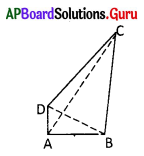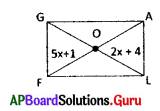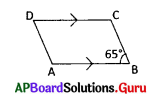Practice the AP 7th Class Maths Bits with Answers Chapter 12 Quadrilaterals on a regular basis so that you can attempt exams with utmost confidence.

## AP State Syllabus 7th Class Maths Bits 12th Lesson Quadrilaterals with Answers

Question 1.
If the diagonals AC, BD of a parallelogram meet at O, then
A) OA = OC
B) OA = OB
C) OA = OD
D) 2 OA = BD
A) OA = OC

Question 2.
ABCD is a parallelogram. ∠A + ∠D =
A) 90°
B) 180°
C) 360°
D) 270°
B) 180°Question 3.
From the given figure, ∠x = ……………….?A) 60°
B) 45°
C) 30°
D) 55°
A) 60°

Question 4.
The sum of the 4 angles of Quadrilateral is ……………………
A) 250°
B) 270°
C) 180°
D) 360°
D) 360°

Question 5.
If ABCD is a rhombus then ……………….
A) AB = CD, BC = AD, AC ≠ BD
B) AB = CD, BC = AD, AC = BD
C) AB = BC = CD = DA, AC ≠ BD
D) AB = BC = CD = DA, AC = BD
C) AB = BC = CD = DA, AC ≠ BD

Question 6.
ABCD is a parallelogram then …………………
A) AB = CD, BC = AD, AC ≠ BD
B) AB = CD, BC = AD, AC = BD
C) AB = BC = CD = DA, AC = BD
D) None
A) AB = CD, BC = AD, AC ≠ BD

Question 7.
Each of a parallelogram, is 90° then it is …………….
A) rhombus
B) square
C) rectangle
D) trapezium
C) rectangle

Question 8.
Three angles of a Quadrilateral are 65°, 120°, 70° then the 4 th angle is ………………..
A) 95°
B) 115°
C) 105°
D) 100°
C) 105°

Question 9.
The angles of a quadrilateral are in the ratio of 1 : 2 : 3 : 4 then the largest angle is ……………….
A) 120°
B) 108°
C) 72°
D) 144°
D) 144°Question 10.
Each angle of a square is …………………
A) 90°
B) 180°
C) 45°
D) 60°
A) 90°

Question 11.
The angles of a quadrilateral are x°, (x + 10°), (x + 20°), (x + 30°) then ∠x = …………….
A) 65°
B) 75°
C) 85°
D) 70°
B) 75°

Question 12.
In a parallelogram ABCD ∠A :∠B = 2 : 3 then ∠C = ………………
A) 90°
B) 108°
C) 72°
D) 60°
C) 72°

Question 13.
Which of the following is true?
A) All rhombuses are parallelograms
B) All kites are rhombuses
C) Every parallelogram is a square
D) Every square is a parallelogram
D) Every square is a parallelogram

Question 14.
A rectangle in which all the sides are equal is called a ……………..
A) parallelogram
B) square
C) rhombus
D) trapezim
B) square

Question 15.
In a rhombus ABCD ∠ADC = 45° then the other angles are ……………….
A) 135°, 45°, 135°
B) 45°, 135°, 135°
C) 60°, 120°, 60°
D) 120°, 60°, 120°
A) 135°, 45°, 135°

Question 16.
A square is a ………………
A) parallelogram
B) rhombus
C) rectangle
D) all the above
D) all the aboveQuestion 17.
If the adjacent angles of a parallelogram are x + 29°, 2x + 31° then ∠x = ……………
A) 30°
B) 40°
C) 60°
D) 120°
B) 40°

Question 18.
Sum of the angles in a quadrilateral in right angles
A) 2
B) 3
C) 4
D) 5
C) 4

Question 19.
Number of independent measurements required for the construction of a quadrilateral
A) 3
B) 5
C) 6
D) 4
B) 5

Question 20.
Sum of the exterior angles of a quadrilateral in right angles
A) 1
B) 2
C) 3
D) 4
D) 4

Question 21.
A quadrilateral which has one pair of opposite sides parallel is called is called a
A) Rhombus
B) Square
C) Parallelogram
D) Trapezium
D) Trapezium

Question 22.
Number of independent measurements for the construction of a trapezium
A) 2
B) 3
C) 4
D) 5
C) 4

Question 23.
The quadrilateral in which the diagonals bisect each other and they are perpendicular to each other
A) Rectangle
B) Rhombus
C) Parallelogram
D) Trapezium
B) RhombusQuestion 24.
The name of the quadrilateral in which the diagonals are equal and perpendicular to each other
A) Parallelogram
B) Rhombus
C) Square
D) Rectangle
C) Square

Question 25.
How many measurements are needed for the construction of a square?
A) 2
B) 3
C) 4
D) 1
D) 1

Question 26.
Measurements (Independent) needed for the construction of a rhombus?
A) 2
B) 3
C) 4
D) 5
A) 2

Question 27.
The number of independent measurements required for the construction of a parallelogram are
A) 2
B) 3
C) 4
D) 5
B) 3

Question 28.
From the given figure given, the diagonals are ………………..A) AB, BC
B) BC, CD
C) AC, BD
C) AC, BD

Question 29.
From the above figure, the angles are
A) ∠A, ∠B
B) ∠D
C) ∠C
D) All the above
D) All the above

Question 30.
From the above figure, the adjacent sides are
A) AB, BC
C) AC, BD
D) AB, CD
A) AB, BC

Question 31.
Two adjacent sides of a parallelogram are in the ratio of 2 : 1. If its perimeter is 21 cm, then its shortest side is ……………… cm
A) 7
B) 6
C) 3.5
D) 3
A) 7Question 32.
ABCD is a square $$\overline{\mathrm{AC}}$$ is the diagonal, then ∠BAC = ……………..
A) 50°
B) 30°
C) 60°
D) 45°
D) 45°

Question 33.
Which of the following is not true?
A) A parallelogram in which two adjacent sides are equal is a rhombus
B) Every diagonal of rectangle divides it into two congruent triangles
C) ABCDisaparallelogram∠A -∠C = 90°.
D) The diagonals of a rhombus bisect at right angles
C) ABCDisaparallelogram∠A -∠C = 90°.

Question 34.
FLAG is a rectangle from the given figure x = …………….. cmA) 1
B) 2
C) 3
D) 4